# 2.模板匹配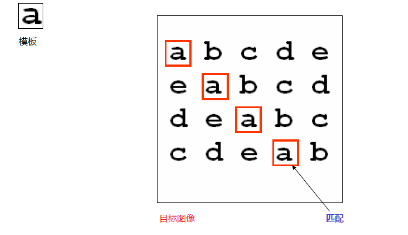• 介绍
• 模板匹配就是在整个图像区域发现与给定子图像匹配的小块区域。
• 所以模板匹配首先需要一个模板图像T（给定的子 图像）
• 另外需要一个待检测的图像-源图像S
• 工作方法，在带检测图像上，从左到右，从上向下计算模板图像与重叠子图像的匹配度，匹配程度越大，两者相同的可能性越大。

# 3.模板匹配介绍——匹配算法介绍

opencv提供了六种常见的匹配算法

• 计算平方不同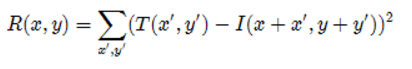• 计算相关性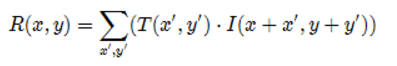• 计算相关系数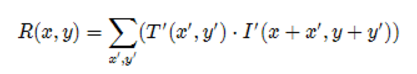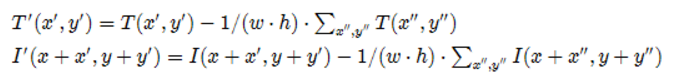（下面归一化的三种方法）
• 计算归一化平方不同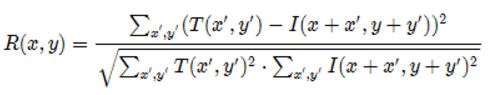• 计算归一化相关性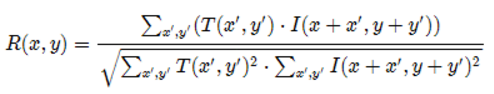• 计算归一化相关系数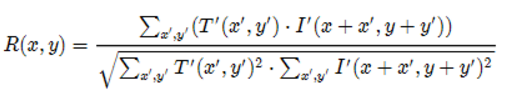上诉公式在opencv中的表示方法# 4.相关API介绍

## 4.1

``````matchTemplate(
InputAurry image,//源图像，必须是8-bit或者32-bit浮点数图像
InputAurry templ,//模板图像，类型必须与输入图像一样
OutputArray result,//输出结果，必须是单通道32为浮点数，
//假设源图像W*H，模板图像w*h，则结果必须为W-w+1，H-h+1的大小
int method,//使用的匹配方法
)
``````

## 4.2 模板匹配的方法

``````enum cv::TemplateMatchModes{
cv::TM_SQDIFF=0;
cv::TM_SQDIFF_NORMED=1;
cv::TM_CCORR=2;
cv::TM_CCORR_NORMED=3;
cv::TM_CCOEFF=4;
cv::TM_CCOEFF_NORMED=5;
}
``````

## 4.3 minMaxLoc（）函数

• 查找全局最小和最大数组元素并返回它们的值和它们的位置。
``````void minMaxLoc(InputArray src,
CV_OUT double* minVal,
CV_OUT double* maxVal=0,
CV_OUT Point* minLoc=0,
CV_OUT Point* maxLoc=0,
);
``````
``````参数1：InputArray类型的src，输入单通道数组（图像）。

``````

# 5.演示代码：

``````void Match_Demo(int,void*){
Mat img_display;
src.copyTo(img_display);
int  result_rows = src.rows - temp.rows + 1;
int  result_cols = src.cols - temp.cols + 1;
dst.creat(Size(result_rows),Size(result_cols ),CV_32FC1);

matchTemplate(src,temp,dst,match_method);
normalize(dst,dst,0,1,NORM_MINMAX,-1,MAT());

double minValue,maxCalue;
Point minLoc;//最小值的位置
Point maxLoc;//最大值的位置
Point matchLoc;

minMaxLoc(dst,&minValue,&maxValue,&minLoc,&maxLoc,MAt());
//此处要区分方法
if(match_method==TM_SQDIFF||match_method==TM_SQDIFF_NORMED){
matchLoc=minLoc;
}
else{
matchLoc=maxLoc;
}

rectangle(img_display,matchloc,Point(matchLoc.x+temp.cols,
matchLoc.y+termp.rows),Scalar::all(0),2,LINE_AA);

rectangle(dst,matchloc,Point(matchLoc.x+temp.cols,
matchLoc.y+termp.rows),Scalar::all(0),2,LINE_AA);

imshow(OUTPUT_T,dst);
imshow(match_t,img_display);
return ;
}
``````

Point类
matchTemplate函数

TM_CCORR匹配出现问题
(原因：这个算法有时候会失真)